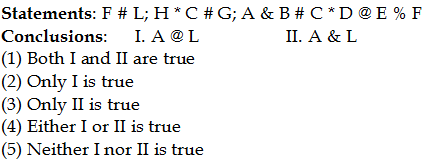## PREPARATORY QUESTIONS

### EQUAL-INEQUAL

#### PREPARATORY SET-18 (HARD)

Direction (Qs.1 to 5): In the following questions, the symbols @, \$, *, # and % are used with the following meaning as illustrated below.

‘P # Q’ means ‘P is smaller than Q’
‘P @ Q’ means ‘P is smaller than or equal to Q’
‘P * Q’ means ‘P is greater than or equal to Q’
‘P % Q’ means ‘P is equal to Q’
‘P & Q’ means ‘P is greater than Q’

Now, in each of the following questions assuming the given statements to be true, find which of the two Conclusions I and II given below them is/are definitely true and give your answer accordingly.

Question No : 1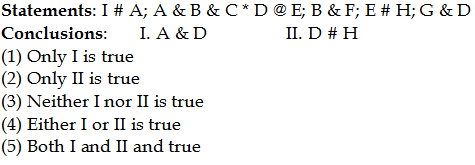Question No : 2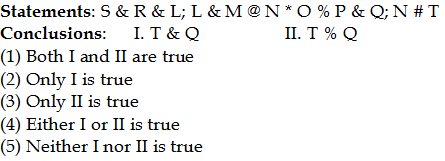Question No : 3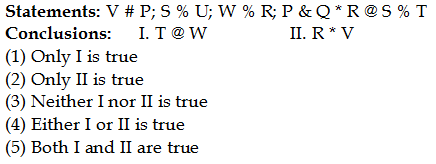Question No : 4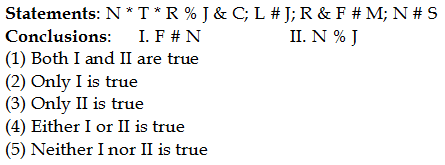Question No : 5